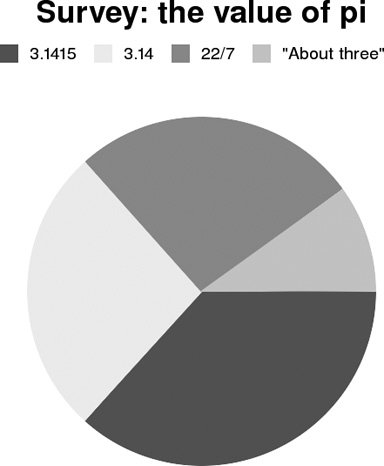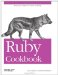# Graphing Data

#### Problem

You want to convert a bunch of data into a graph; usually a line chart, bar chart, or pie chart.

#### Solution

Use the Gruff library, written by Geoffrey Grosenbach. Install the gruff gem and build a Gruff object corresponding to the type of graph you want (for instance, Gruff::Line, Gruff::Bar,or Gruff::Pie). Add a dataset to the graph by passing data a label and an array of data points.

Heres code to create a graph that compares the running times of different sorts of algorithms:

```	require
ubygems
require gruff

g = Gruff::Line.new(600) # The graph will be 600 pixels wide.
g.title = Algorithm running times
g.theme_37signals # The best-looking theme, in my opinion.

range = (1..101)
g.data(Constant, range.collect { 1 })
g.data(O(log n), range.collect { |x| Math::log(x) / Math::log(2) })
g.data(O(n), range.collect { |x| x })
g.data(O(n log n), range.collect { |x| x * Math::log(x) / Math::log(2) })

g.labels = {10 =>
=10, 50 =>
=50, 100 =>
=100 }
g.write(algorithms.png)
```

Figure 12-3 shows the graph it produces.

##### Figure 12-3. A line chartHeres code to create a pie chart (shown in Figure 12-4). Note that the numbers given for the datasets don have to add up to 100. Gruff automatically scales the the pie chart to display the right proportions.

```	p =
Gruff::Pie.new
p.theme_monochrome
p.title = "Survey: the value of pi"
p.data(3.14, )
p.data(3.1415, )
p.data(22/7, )

p.write(pipie.png)
```

##### Figure 12-4. A pi chart#### Discussion

Most of the time, programmers who need a graphing library need a simple graphing library: one that lets them easily produce a quick pie, line, or bar graph. Gruff works well for graphing simple datasets, but it doesn have the functionality of a fullfledged math program.

Gruffs interface for customizing the display of datasets also leaves something to be desired. Instead of letting you tweak the colors individually, it provides a number of themes that package together a background image, a text color, and a number of colors used in the graphs. Unfortunately, most of the provided themes are ugly (theme_37signals is pretty nice, though).

Heres a custom theme that makes monochrome graphs whose "colors" can be fairly easily distinguished. It takes advantage of the fact that its easy to distinguish dark shades of gray from light shades, and that lighter shades are more easily distinguishable from one another. The graphs in this recipe were actually created with this theme_monochrome, so that the "colors" would be more easily distinguishable in a printed book.

```	class
Gruff::Base
def theme_monochrome
reset_themes
@colors = "6E9C7ADB".scan(/./).collect { |c| "##{c * 6}"}
@marker_color = lack
@base_image = render_gradiated_background(white, white)
end
end
```

This code adds writer methods for the various colors, letting you modify the current theme on an ad hoc basis. colors sets the colors used to differentiate datasets from each other. marker_color method sets the color of the title and axis labels. background sets the background to a solid color, or to a gradient between two colors.

```	class
Gruff::Base
def colors=(colors)
@colors = colors
end

def marker_color=(color)
@marker_color = color
end

def background=(color1, color2=nil)
color2 ||= color1
@base_image = render_gradiated_background(color1, color2)
end
end
```

• The Gruff homepage (http://nubyonrails.topfunky.com/pages/gruff)
• A couple of other Ruby graphing libraries deserve mention:

• MRPlot is useful for plotting mathematical functions; its default implementation works on top of RMagick (http://harderware.bleedingmind.com/index.php?l=en&p=mrplot)
• The SVG::Graph library doesn need any external libraries and produces beautiful SVG graphs; unfortunately, not many programs have support for SVG graphics, although newer versions of Firefox do (http://www.germane-software.com/software/SVG/SVG::Graph/)Ruby Cookbook (Cookbooks (OReilly))
ISBN: 0596523696
EAN: 2147483647
Year: N/A
Pages: 399